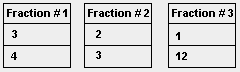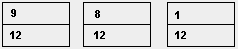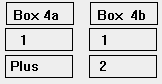Fraction Calculator For Addition or Subtraction I N S T R U C T I O N S This calculator can add or subtract up to 5 fractions at once and only has a few rules: * * * Denominators can't be zero. * * * Denominators can't be greater than 25. * * * Each fraction must have both the numerator and denominator box filled in, otherwise this creates an error message and nothing is calculated. For practice, input   3/4   2/3   and   1/12  as shown:then click CALCULATE. The 2 rows beneath the calculate button will show these 3 fractions converted to fractions with common denominators:Box 1 shows the numerators over the common denominator and Boxes 2 and 3 show the Box 1 fraction being reduced.The Box 3 fraction is then split into a whole number (Box 4a) plus its fractional part (Box 4b), which in this example equals 1 ½So, this calculator determines the common denominator, shows what each fraction becomes, shows the sum of the numerators over the denominator, reduces the fraction to simplest terms and puts the fraction into the "whole number plus a fraction" form. When subtracting a fraction, put a minus sign on the numerator or denominator - but not BOTH. NOTE: If the answer is less than zero, boxes 4a and 4b will NOT be filled in due to the confusion caused by trying to show 1 negative number in 2 boxes. Good luck with those fractions !!! Return To Home Page Copyright © 1999 - 1728 Software Systems